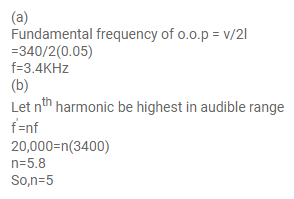# An open organ pipe has a length of`
Question:

An open organ pipe has a length of $5 \mathrm{~cm}$.

(a) Find the fundamental frequency of vibration of this pipe.

(b) What is the highest harmonic of such a tube that is in the audible range? Speed of sound in air is $340 \mathrm{~m} \mathrm{~s}^{-1}$ and the audible range is $20-20,000$ $\mathrm{Hz}$.

Solution: